﻿ 搅拌摩擦焊缝涡流检测

# 搅拌摩擦焊缝涡流检测Eddy Current Testing of Friction Stir Welds

Abstract: In the process of friction stir welding (FSW), due to the improper selection of process parameters and the design of the stir head, there will be welding defects. In this paper, a three-dimensional motion mechanical device is designed to carry out C-scan for friction stir welding to realize eddy current detection. ANSYS and Adams are used to simulate the ball screw, and the transient load and its linear relationship are analyzed. In order to improve the ability of defect detection, only by analyzing the characteristics of differential detection signal, and then obtaining the detected defect information, this paper designs a cone-shaped pulsed eddy current sensor, and analyzes its magnetic field. The electromagnetic simulation was carried out by COMSOL, and the magnetic field distribution images of aluminum specimen with and without defects were compared with those with or without shield cover. Based on the magnetic shielding existing in ferromagnetic materials, the ferromagnetic simulation experiment was carried out. Then, the ant colony algorithm is used for contour recognition, and singular value decomposition (SVD) denoising, Kalman filtering and RBF optimized Kalman filtering are carried out successively. Finally, VB man-machine interface is established for effective control.

1. 引言

2. 脉冲涡流检测原理

2.1. 涡流效应原理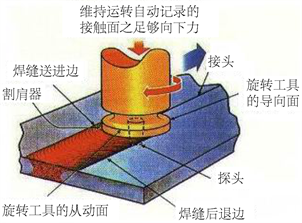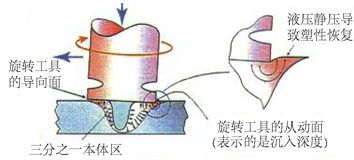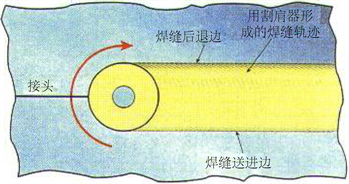Figure 1. Working principle of friction stir welding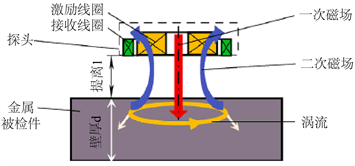Figure 2. Principle of eddy current effect

2.2. 趋肤效应原理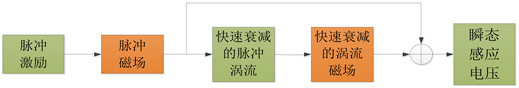Figure 3. Basic principle of pulsed eddy current testing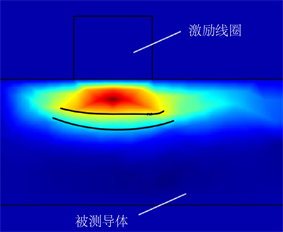(a) 导体电磁特征连续的涡流分布(b) 导体电磁特征不连续

Figure 4. Distribution of induced eddy current

2.3. 提离效应原理

3. 搅拌摩擦焊涡流机械装置

3.1. 三维运动平台设计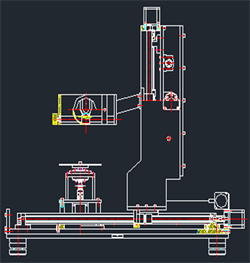Figure 5. 3D motion platform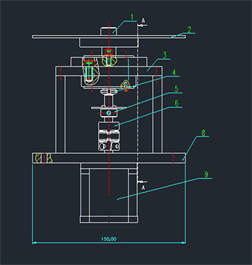Figure 6. Design of rotating platform

3.2. 滚珠丝杠运动控制方案设计

3.3. ANSYS滚珠丝杠瞬态分析Figure 7. Ball screw motion control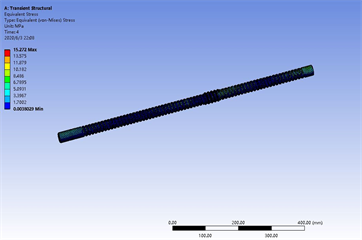Figure 8. Transient analysis of ANSYS ball screw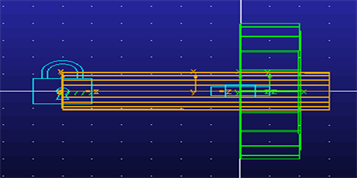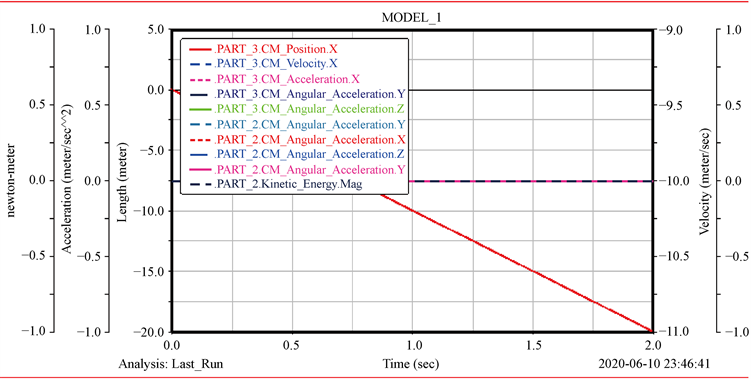Figure 9. Simulation of Adams ball screw

4. 圆台状脉冲涡流差分传感器

4.1. 圆台状脉冲涡流差分传感器

4.2. 圆台状差分传感器磁场解析模型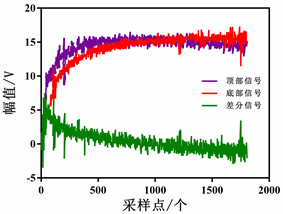(a) 差分信号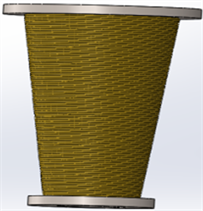(b) 圆台状差分传感器线圈

Figure 10. Cone shaped differential sensor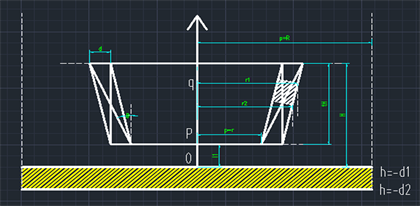Figure 11. Model of conical coil above finite thickness conductor

${A}_{pk}=\underset{i=1}{\overset{\infty }{\sum }}K{{C}^{\prime }}_{3,i}\left({\text{e}}^{{\lambda }_{0i}h}+{{F}^{\prime }}_{0,i,li}{\text{e}}^{-{\lambda }_{0i}h{\text{e}}^{-2{\lambda }_{0}{d}_{1}}}\right)$ (1)

${A}_{qk}=\underset{i=1}{\overset{\infty }{\sum }}K{{C}^{\prime }}_{1,i}\left({\text{e}}^{{\lambda }_{0i}h}+{{C}^{\prime }}_{3,i}{{F}^{\prime }}_{0,i,li}{\text{e}}^{-{\lambda }_{0i}h{\text{e}}^{-2{\lambda }_{0}{d}_{1}}}\right)$ (2)

${{C}^{\prime }}_{1,i}={\text{e}}^{{\lambda }_{0i}\left({l}_{1}+k\cdot \Delta H\right)}-{\text{e}}^{{\lambda }_{0}\left({l}_{1}+k\cdot \Delta H-\Delta H\right)}$ (3)

${A}_{p}=\underset{k=1}{\overset{N}{\sum }}{A}_{pk}=\underset{k=1}{\overset{N}{\sum }}\underset{i=1}{\overset{\infty }{\sum }}K{{C}^{\prime }}_{3,i}\left({\text{e}}^{{\lambda }_{0i}h}+{{F}^{\prime }}_{0,i,li}{\text{e}}^{-{\lambda }_{0i}h{\text{e}}^{-2{\lambda }_{0}{d}_{1}}}\right)$ (4)

${A}_{q}=\underset{k=1}{\overset{N}{\sum }}{A}_{qk}=\underset{k=1}{\overset{N}{\sum }}\underset{i=1}{\overset{\infty }{\sum }}K{{C}^{\prime }}_{1,i}\left({\text{e}}^{{\lambda }_{0i}h}+{{C}^{\prime }}_{3,i}{{F}^{\prime }}_{0,i,li}{\text{e}}^{-{\lambda }_{0i}h{\text{e}}^{-2{\lambda }_{0}{d}_{1}}}\right)$ (5)

${B}_{ph}=\frac{1}{\rho }\frac{\partial }{\partial \rho }\left(\rho {A}_{p}\right)=\underset{k=1}{\overset{N}{\sum }}\underset{i=1}{\overset{\infty }{\sum }}\frac{\alpha {\mu }_{0}{i}_{0}\chi \left({\lambda }_{0i}{r}_{1k},{\lambda }_{0i}{r}_{2k}\right){{C}^{\prime }}_{3,i}\left({\text{e}}^{{\lambda }_{0i}h}+{{F}^{\prime }}_{0i,li}{\text{e}}^{-{\lambda }_{0i}h}\right)\left[{J}_{1}\left({\lambda }_{0i}\rho \right)+{\lambda }_{0i}\rho {{J}^{\prime }}_{1}\left({\lambda }_{0i}pp\right)\right]}{{\left[\left({\lambda }_{0i}R\right){J}_{0}\left({\lambda }_{0i}R\right)\right]}^{2}\rho {\lambda }_{0i}^{2}}$ (6)

${B}_{qh}=\frac{1}{\rho }\frac{\partial }{\partial \rho }\left(\rho {A}_{q}\right)=\underset{k=1}{\overset{N}{\sum }}\underset{i=1}{\overset{\infty }{\sum }}\frac{\alpha {\mu }_{0}{i}_{0}\chi \left({\lambda }_{0i}{r}_{1k},{\lambda }_{0i}{r}_{2k}\right)\left({{C}^{\prime }}_{3,i}{\text{e}}^{-{\lambda }_{0i}h}+{{C}^{\prime }}_{3,i}{{F}^{\prime }}_{0i}\right)\left[{J}_{1}\left({\lambda }_{0i}\rho \right)+{\lambda }_{0i}\rho {{J}^{\prime }}_{1}\left({\lambda }_{0i}\rho \right)\right]}{{\left[\left({\lambda }_{0i}R\right){J}_{0}\left({\lambda }_{0i}R\right)\right]}^{2}\rho {\lambda }_{0i}^{2}}$ (7)

4.3. 有限元仿真研究

4.3.1. 有限元模型的建立

(1) 几何建模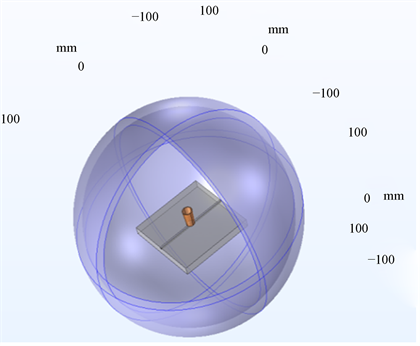Figure 12. Simulation model of defect testing for non ferromagnetic materials

(2) 设定参数

$\left(J\omega \sigma -{\omega }^{2}\epsilon \right){A}_{\phi }+\nabla ×\left({\mu }^{-1}\nabla ×{A}_{\phi }\right)={J}_{\phi }^{e}$ (8)

$-\left(\nabla JV+\frac{\partial {A}_{\phi }}{\partial t}\right)=\sigma \epsilon ={J}_{\phi }^{e}$ (9)

$H=\frac{B}{\mu }=\frac{\nabla ×{A}_{\phi }}{{\mu }_{0}{\mu }_{r}}$ (10)

(3) 网格划分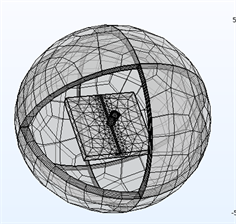Figure 13. Finite element mesh generation of non ferromagnetic simulation model

4.3.2. 检测计算灵敏度计算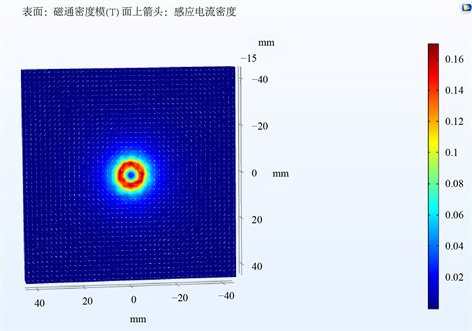(a) 无缺陷铝试件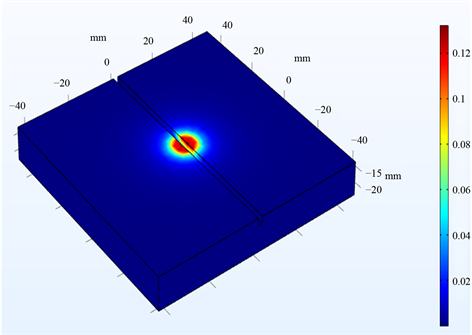(b) 有缺陷铝试件

Figure 14. Distribution of eddy current density on the surface defects of non ferromagnetic metal samples

$\frac{\Delta {B}_{e}}{\Delta V}=S$ (11)

4.3.3. 铁磁屏蔽抗干扰技术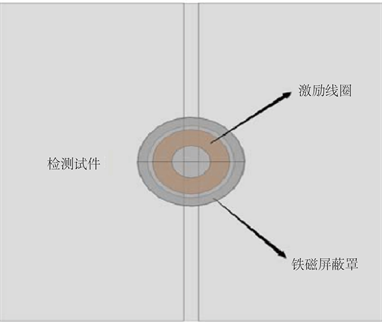Figure 15. Structure of ferromagnetic shield

4.3.4. 铁磁仿真试验研究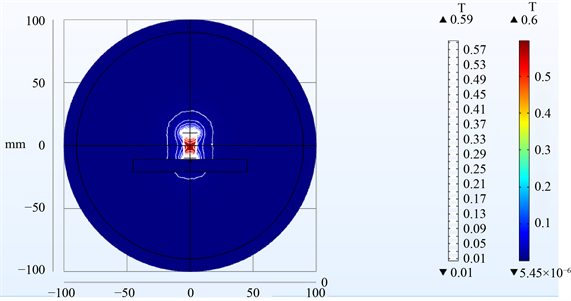(a) 铁磁屏蔽线圈的磁场分布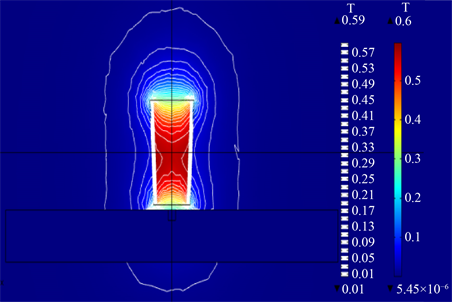(b) 铁磁屏蔽线圈的磁场分布局部图

Figure 16. Magnetic field distribution of unloaded shield coil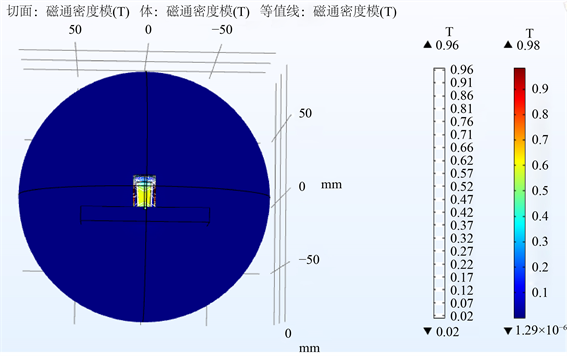(a) 没有加载铁磁屏蔽罩后磁场分布整体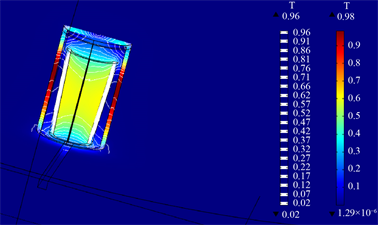(b) 没有铁磁加载屏蔽罩后磁场分布局部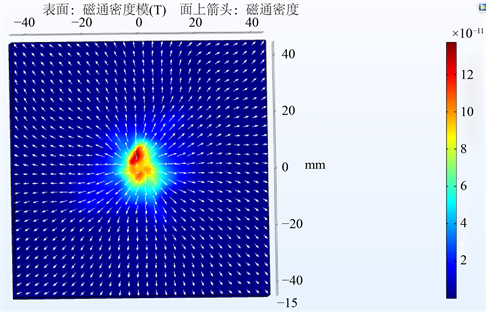(a) 铁磁(铁)试件表面在圆台状探头面的磁场分布图(0.02s)(b) 非铁磁性(铝)试件表面在圆台状探头面的磁场分布图(时间0.02s)

Figure 18. Distribution of material permeability vs. surface flux density and eddy current

5. 缺陷轮廓识别

5.1. 脉冲涡流扫描介绍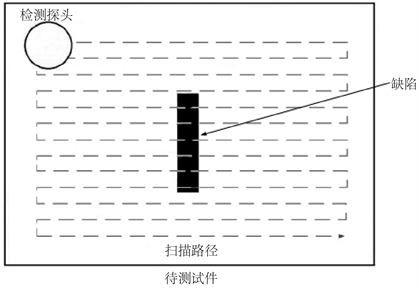Figure 19. Path scan

5.2. 蚁群算法识别轮廓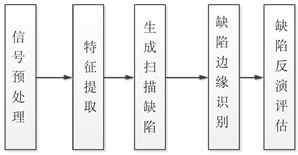Figure 20. Steps of automatic defect assessment method

(1) 信号预处理：选择连续10个周期的脉冲涡流瞬态响应信号，并取其平均值以消除一些高频噪声；

(2) 特征提取：将每个采样位置所得的脉冲涡流瞬态响应信号，利用基于Fisher判别和系统识别法的在线缺陷识别分类法，提取特征量，反映缺陷信息的特征值，然后生成扫描成像图。创建一个矩阵 ${F}^{m×n}$，其中m和n分别为扫描区域X方向和Y方向所采样的点数。通过基于Fisher判别的在线缺陷识别分类方法和系统识别方法，将从每个采样位置获得的脉冲涡流瞬态响应信号用于提取特征量并反映缺陷信息的特征值，然后进行扫描成像图。创建一个矩阵 ${F}^{m×n}$，其中m和n分别为扫描区域X方向和Y方向所采样的点数；

(3) 缺陷边缘检测：边缘检测算法用于提取缺陷的边缘并确定缺陷所在的位置。依靠获得的缺陷边缘信息，获得探针在缺陷上方时采集的脉冲涡流瞬态响应信号，并采用基于蚁群算法的缺陷边缘检测方法进行缺陷参数自动识别。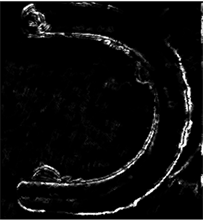Figure 21. Identification results of ant colony algorithm

6. 脉冲涡流信号的预处理

6.1. 奇异值分解去噪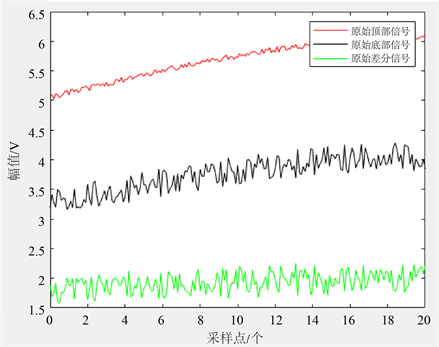(a) 拟定的差分信号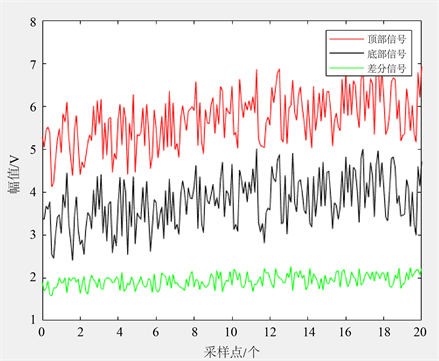(b)奇异值分解去噪

Figure 22. Differential signal after singular value processing

6.2. 卡尔曼滤波

6.3. RBF优化卡尔曼滤波Figure 23. Kalman filter

6.4. 人机操作界面的设计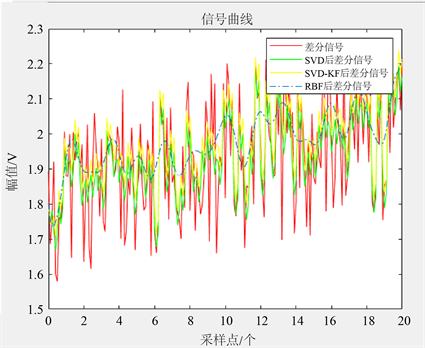Figure 24. RBF optimized Kalman filterFigure 25. VB man machine interface

7. 结论

 Ma, Z.Y. (2008) Friction Stir Processing Technology: A Review. Metallurgical and Materials Transactions A, 39, 642-658.
https://doi.org/10.1007/s11661-007-9459-0

 栾国红, 郭德伦, 张田仓, 等. 搅拌摩擦焊在飞机制造工业中的应用[J]. 航空制造技, 2002(11): 11-20.

 Takeshi, S. (2001) Recent Development of a Friction Stir Welding New Solid State Joining Technology. International Journal of Materials and Product Technology, 2, 453-456.

 王快社, 王训宏, 沈洋, 等. 搅拌摩擦焊技术的最新进展[J]. 热加工工艺, 2004(10): 53-55.

 周万盛, 姚君山. 铝及铝合金的焊接[M]. 北京: 机械工业出版社, 2006.

 Kinchen, D.G. and Aldahir, E. (2002) NDE of Friction Stir Welds in Aerospace Applications. Lockheed Martin Michoud Space Systems, New Orleans, 1-7.

 Ofune, M.D., et al. (2016) Development of Valve Train Rig for Assessment of Cam/Follow Tribochemistry. Tribology International, 93, 733-744.
https://doi.org/10.1016/j.triboint.2015.02.026

 Mahaut, S., Jenson, F., Calmon, P., Darmon, M. and Chatillon, S. (2009) Recent Advances and Current Trends of in CIVA. Insight-Non-Destructive Testing and Condition Monitoring, 51, 78-81.
https://doi.org/10.1784/insi.2009.51.2.78

 Sophian, A. (2003) Characterization of Surface and Sub-Surface Discontinuities in Metals Using Pulsed Eddy Current Sensors. The University of Huddersfield in Partial Fulfillment of the Requirements for the Degree of Doctor of Philosophy, The United Kingdom.

 Zhang, J., He, K., Zheng, X., et al. (2010) An Ant Colony Optimization Algorithm for Image Edge Detection. Internal Conference on Artification Intelligence and Compulation Intelligence, Sanya, 23-24 October 2010, 215-219.
https://doi.org/10.1109/AICI.2010.167

 王俊, 周德强, 肖俊峰, 等. 铁磁性材料脉冲涡流检测信号的消噪方法[J]. 无损检测, 2016, 38(1): 44-48.

 Hassanpour, H., Zehtabian, A. and Sadati, S.J. (2012) Time Domain Signal Enhancement Based on an Optimized Singular Vector Denoising Algorithm. Digital Signal P, 22, 786-794.
https://doi.org/10.1016/j.dsp.2012.03.009

 宋文尧. 卡尔曼滤波[M]. 北京: 科学出版社, 1991.

 田景文, 高美娟. 人工神经网络算法研究及应用[M]. 北京: 北京理工大学出版社, 2006.

Top